Auto-Encoding Variational Bayes

In my previous post on variational inference, I gave a quick foundation of the theory behind variational auteoncoders (VAEs). The dominant lay understanding of VAEs is that they’re models which allow you to take map an image to a smaller latent space and manipulate that image in meaningful ways by changing the values of the latent space. Most machine learning tutorials are centered around this interpretation. However, I feel this short-sells their meaning (and makes people say “just use a GAN instead”, which is disappointing). It is more correct, and interesting, to say that VAEs are an implementation of variational inference which allows use of arbitrarily complex functions (read: neural networks) as approximating distributions.

Like the previous post, a lot of this information was pulled from the Stanford Probabilistic Graphical Models Course, but it’s also worth reading the original (if opaque) Kingma & Welling Paper, watching this video (starting at 7 minutes or so), and reading this StackOverflow post.

Throughout this post,refers to an input,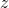refers to latent variables,refers to an actual distribution, andrefers to an approximating distribution.

In a variational autoencoder, we have a (true) equation, and want to estimate our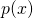.

In order to do this, the first thing we do is re-formulate the VAE objective. We still use the upper bound:Which, in our variables, is:We note that KL is always greater than or equal to zero, so we remove the intractable first KL term. We then expand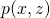with the probability chain rule (and logarithm product rule).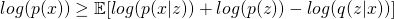Exploit the linearity of expectation: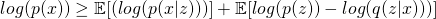Flip the signs and collect the right two terms as a KL divergence (asmarginalizes out, this is a straightforward algebraic manipulation of the KL divergence):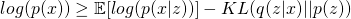Which is our objective function for the variational autoencoder. We can pause here for a minute and take a look at how this lines up with the common interpretation of our variational autoencoder. We note that there is a, which corresponds to our encoder network, a, which corresponds to our prior (a set of independent multivariate unit gaussians), and a, which corresponds to our decoder network. The expected value operator is also significant, as it means we never actually need to know the value of the probability. The expected value is simply the output of the decoder network when all latent variables are at their mean value, and making the probability ofhigher under this distribution corresponds to just making the input and output images match more closely!

There is one (not small) detail missing before we can perform gradient descent. Sinceare random variables, we can’t differentiate through them to train the encoder and decoder network. This is what the reparameterization trick is for. In the reparameterization trick, we produce two outputs in our latent space (mean and standard deviation) and treat them as deterministic. That is, these are the outputs we want to learn. But we have a third, random, variable that we multiply sigma by which is drawn from a unit normal distribution. Since this isn’t learned, we can still backpropagate through the network as desired despite having random variables within it.

This site uses Akismet to reduce spam. Learn how your comment data is processed.